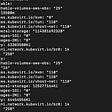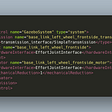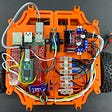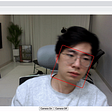# Exploring Elliptic Curve Pairings

`a + b:  (a + b) % pa * b:  (a * b) % pa - b:  (a - b) % pa / b:  (a * b^(p-2)) % p`
`2 + 3 = 5 % 7 = 54 + 6 = 10 % 7 = 32 - 5 = -3 % 7 = 46 * 3 = 18 % 7 = 43 / 2 = (3 * 2^5) % 7 = 55 * 2 = 10 % 7 = 3`
`(2 + 3i) + (4 + 2i) = 6 + 5i(5 + 2i) + 3 = 1 + 2i(6 + 2i) * 2 = 5 + 4i4i * (2 + i) = 3 + i`
`a / b:  (a * b^(p^2-2)) % p`
• G1 is an elliptic curve, where points satisfy an equation of the form y² = x³ + b, and where both coordinates are elements of F_p (ie. they are simple numbers, except arithmetic is all done modulo some prime number)
• G2 is an elliptic curve, where points satisfy the same equation as G1, except where the coordinates are elements of F_p¹² (ie. they are the supercharged complex numbers we talked about above; we define a new “magic number” w, which is defined by a 12th degree polynomial like `w^12 - 18 * w^6 + 82 = 0`)
• Gt is the type of object that the result of the elliptic curve goes into. In the curves that we look at, Gt is F_p¹² (the same supercharged complex number as used in G2)
• e(P, Q + R) = e(P, Q) * e(P, R)
• e(P + Q, R) = e(P, R) * e(Q, R)
• Efficient computability (eg. we can make an easy pairing by simply taking the discrete logarithms of all points and multiplying them together, but this is as computationally hard as breaking elliptic curve cryptography in the first place, so it doesn’t count)
• Non-degeneracy (sure, you could just define e(P, Q) = 1, but that’s not a particularly useful pairing)
• The function is equal to zero at P, since x is P_x, so x - P_x = 0
• The function is equal to zero at -P, since -P and P share the same x coordinate
• The function goes to infinity as x goes to infinity, so we say the function is equal to infinity at O. There’s a technical reason why this infinity needs to be counted twice, so O gets added with a “multiplicity” of -2 (negative because it’s an infinity and not a zero, two because of this double counting).
• (F_P) = n * [P] - n * [O], where n is the order of G1, ie. n * P = O for any P
• (F_Q) = n * [Q] - n * [O]
• (g) = [P + Q] - [P] - [Q] + [O]

--

--

--

## More from Vitalik Buterin

Love podcasts or audiobooks? Learn on the go with our new app.

## Creating a HTTP Server in Go using gin## 5 Easy Things to fix Machine Learning## Easy ABAP Business Application Log (BAL) with the Interface IF_RECA_MESSAGE_LIST## How to build a successful software organization inside a larger one## 4 Easy Tips for Using Threads and Mutexes in C++## Platform vs. Point Solutions — Solution Flexibility and the Last 20%## A Complete 12-Week Course to Learn Web Scraping in Python for Free## A try to migrate virtual machine from Openstack to Red Hat Openshift Virtualization## Robot Operating System: Expose Control Nodes for an Interactive Simulation in Gazebo## Building the ROS Robot: Chassis, Wiring, and Safety## Legged robots: ROS2## WASM and SIMD with a sample (C++, TFLite)